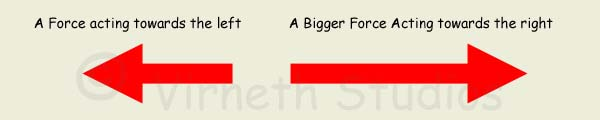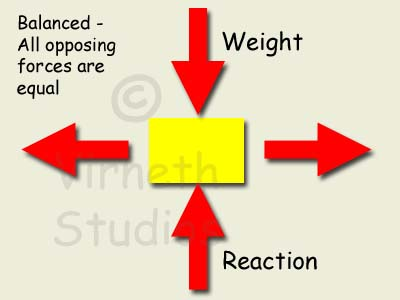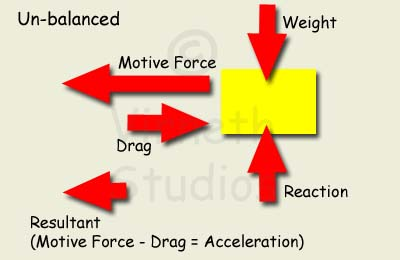# Forces

## What is a Force?

A Force is an action that changes the movement of something; it is a PUSH or a PULL. A Force is measured in Newtons.

We represent a force as an arrow. The length of the arrow shows how big the force is and the arrow points in the direction the force is acting. When we have both a size (magnitude) and a direction it is called a VECTOR.### Vectors and Scalars

A Scalar is a quantity with only a magnitude, Examples would include mass, speed, length, area, volume, density, mass, temperature, power, work, pressure, energy and entropy.

A Vector is a quantity with magnitude and direction. Examples would include force, velocity, acceleration, momentum, lift, drag, weight, thrust and displacement.

When observing the world around us you will notice that there are some quantities that do not depend on the direction and some that do. For example the quantity mass is not direction dependent, it does not matter which direction it is going; a 1kg mass is still a 1kg mass going up or down. On the other hand it does matter which way weight is going, up is different from down.

We differentiate between Scalars and Vectors by giving Vectors a + or - sign, we can then add them up i.e. +5 +(-3N) = +2N, the result still has a direction. A Scalar is just a quantity and always positive. There is no such thing as -1kg of mass, it would be non-existent!

### Force Diagrams

The concept of Vectors allow us to define how forces interact with each other and to find the result. This is commonly done using a force diagram.

In a Force diagram we show arrows representing the forces. The length of each arrow is proportional to the magnitude (size) of the force.## Laws of Motion

Sir Isaac Newton devised three laws to explain the principle of Forces and Motion:

## The First Law of Motion - Balanced forces equals no change

### "A body will remain in a state of rest or uniform motion (constant velocity) in a straight line unless that state is changed by an external unbalanced force acting upon it."

When something is moving constantly or is stationary then the forces acting on it are Balanced - there is no change of momentum. Momentum refers to a  moving objects tendency to keep going I.e. a car will coast even when the accelerator has been lifted - (in reality the car will decelerate (a negative acceleration) because the air resistance and ground friction will slow it down).

Momentum = Mass x Velocity.

The object must be moving with constant velocity in a straight line for the forces to be balanced. Velocity is the measure of how much distance a body has moved in a specified amount of time; it is another vector as it has both a size and a direction. Any change in velocity (its size or its direction) is an Acceleration (another vector) and will be because the forces have become unbalanced.

For example when a car moves along steadily, the force driving it must be equal to the force (drag/friction) opposing it, and the upthrust from the ground must be equal to the objects weight.

## The Second Law of Motion - Unbalanced Forces Result in Acceleration

### "Force is equal to the change in momentum over change in time. For a constant mass, Force = mass x acceleration."

F = (m x v2) - (m x v1)

F = m(v2-v1)

F = m.a

When forces are unbalanced they cause an object to change direction, speed up or slow down. Unbalanced forces always change velocity and any change in velocity (either magnitude or direction) is an acceleration.## The Third Law of Motion - Action and Reaction

### "For every action there is an equal and opposite re-action."

This means that if you push on an object then the object pushes back with an equal but opposite force.

For example if you push against a wall the wall pushes back with the same amount of force, if it didn't it would fall over...similarly, if a cleaning service scrubs a floor, the floor pushes back with the same force otherwise the cleaners would fall through the floor.

When you hold a coin in your hand, it's weight exerts a downward force on your hand. To stop it moving, your hand exerts an equal force upwards.

The Jet Engine uses Newton's Third Law of Motion - the force of hot gases accelerating out of the back create a Reactive force pushing the engine forward.